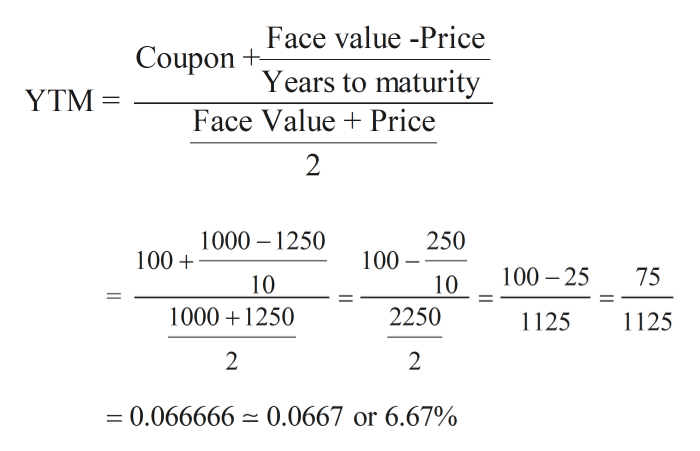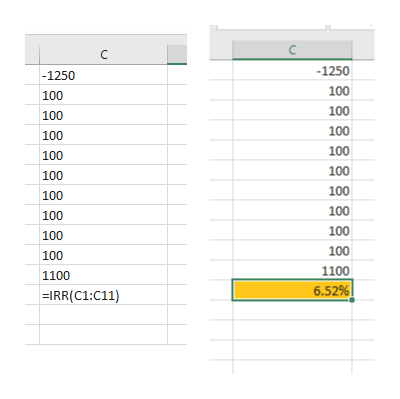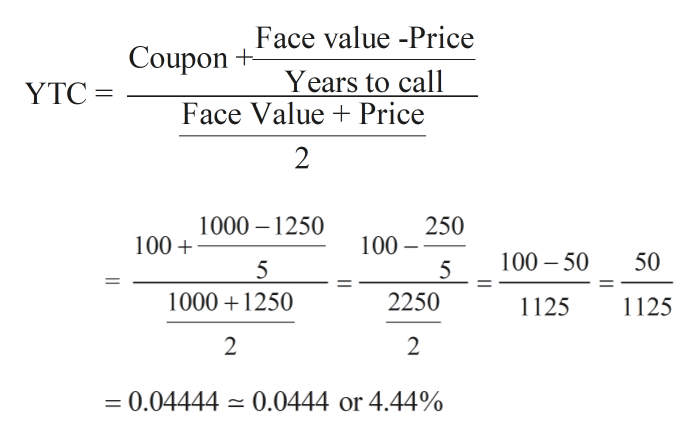Yield to Maturity and Yield to CallArnot International's bonds have a current market price of \$1,250. The bonds have an 10% annual coupon payment, a \$1,000 face value, and 10 years left until maturity. The bonds may be called in 5 years at 109% of face value (call price = 1,090). What is the yield to maturity? Round your answer to two decimal places.What is the yield to call if they are called in 5 years? Round your answer to two decimal places.Which yield might investors expect to earn on these bonds, and why?-Select-IIIIIIIV I. Investors would not expect the bonds to be called and to earn the YTM because the YTM is greater than the YTC.II. Investors would not expect the bonds to be called and to earn the YTM because the YTM is less than the YTC.III. Investors would expect the bonds to be called and to earn the YTC because the YTC is less than the YTM.IV. Investors would expect the bonds to be called and to earn the YTC because the YTM is less than the YTC.The bond's indenture indicates that the call provision gives the firm the right to call them at the end of each year beginning in Year 5. In Year 5, they may be called at 109% of face value, but in each of the next 4 years the call percentage will decline by 1 percentage point. Thus, in Year 6 they may be called at 108% of face value, in Year 7 they may be called at 107% of face value, and so on. If the yield curve is horizontal and interest rates remain at their current level, when is the latest that investors might expect the firm to call the bonds?-Select-In Year 6In Year 7In Year 8In Year 9Bonds are always called

Question

Yield to Maturity and Yield to Call

Arnot International's bonds have a current market price of \$1,250. The bonds have an 10% annual coupon payment, a \$1,000 face value, and 10 years left until maturity. The bonds may be called in 5 years at 109% of face value (call price = 1,090).

1. What is the yield to maturity? Round your answer to two decimal places.

2. What is the yield to call if they are called in 5 years? Round your answer to two decimal places.

3. Which yield might investors expect to earn on these bonds, and why?
-Select-IIIIIIIV
I. Investors would not expect the bonds to be called and to earn the YTM because the YTM is greater than the YTC.
II. Investors would not expect the bonds to be called and to earn the YTM because the YTM is less than the YTC.
III. Investors would expect the bonds to be called and to earn the YTC because the YTC is less than the YTM.
IV. Investors would expect the bonds to be called and to earn the YTC because the YTM is less than the YTC.

The bond's indenture indicates that the call provision gives the firm the right to call them at the end of each year beginning in Year 5. In Year 5, they may be called at 109% of face value, but in each of the next 4 years the call percentage will decline by 1 percentage point. Thus, in Year 6 they may be called at 108% of face value, in Year 7 they may be called at 107% of face value, and so on. If the yield curve is horizontal and interest rates remain at their current level, when is the latest that investors might expect the firm to call the bonds?
-Select-

In Year 6

In Year 7

In Year 8

In Year 9

Bonds are always called

Step 1

First three subparts will be answered as per our policies.

1)

Coupon = 10% of Face value = 10% of 1000 = 100

Yield to maturity (YTM) can be calculated by using the approximation formula or by using Excel function IRR.

YTM calculation using approximation formula is shown below.help_outlineImage TranscriptioncloseFace value -Price Coupon Years to maturity Face Value Price YTM 2 1000 1250 250 100 100 100 25 75 10 10 1000 1250 2250 125 1125 2 2 = 0.066666 0.0667 or 6.67% fullscreen
Step 2

YTM calculation using excel can be done using IRR function as shown below.help_outlineImage TranscriptioncloseC -1250 -1250 100 100 100 100 100 100 100 100 100 100 100 100 100 100 100 100 100 100 1100 1100 6.52% | =IRR(C1:C11) fullscreen
Step 3

2)

For calculating Yield to call (YTC), same formulae can be used with a minor modificati...help_outlineImage TranscriptioncloseFace value -Price Coupon+ Years to call Face Value Price YTC 1000 1250 100 250 100 5 100 50 50 5 10001250 2250 1125 1125 2 2 - 0.04444 0.0444 or 4.44% 11 fullscreen

Want to see the full answer?

See Solution

Want to see this answer and more?

Our solutions are written by experts, many with advanced degrees, and available 24/7

See Solution
Tagged in